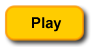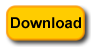Breaking Down Large PHP Problems into Classes: A Class Should Only have a Single ResponsibilityA Little UX and HCI

I’ve been working with a student doing experiments based on Gestalt psychology and User Experience (UX) and Human Computer Interaction (HCI). She wanted to find out whether the Gestalt concepts of similarity and proximity could be applied to a user interface (UI). So she set up an experiment to see what happened.

In summary, she tested using UIs with similarity/no similarity and proximity/no proximity. The subjects were given a task that would test each condition and their times were compared. After each test, the times were saved in a MySQL database and then retrieved to compare the mean times (average amount of time) that each task took. As predicted, the raw data showed that when the Gestalt principles were observed, the task took less time than when they were not.

While just looking at the data showed a difference, she did not know whether the differences were significant. The most appropriate way to find whether the differences are significant is to conduct a statistical test called a “means test” or “t-test” that compares two means. The t-test would tell us with a precise degree of probability whether the differences were random or not. Use the following buttons to view the outcome and download the files:The T-TestFigure 1: The t-test

Figure 1 shows the statistic used for the t-test. Let’s break down the t-test:

• t: This is the value generated by the t-test. Using it, we use a statistical look-up table to see to what degree this is significant or not. (Because the sample size is 50, the degrees of freedom is 49, and so the t value must be 3.2651 or greater for the difference to be significant at the .001 level of significance. That means there’s about 1 chance in 1000 that these differences would be random.)
• x1, x2: The x1 and x2 values are the means. This value is easy to calculate. You just divide the total of the values in the array using the function array_sum(\$array) by the number of cases–count(\$array). (In the diagram you see the x’es with a line over them and the numbers are subscripted.)
• Triangle: The triangle (or delta) is the symbol used for difference in the samples. The null hypothesis is that there will be no differences, and so the value of delta is zero.
• s1,s2: The s values are standard deviations. These are the amount of differences between the observed value and the mean. In the formula, the s values are squared–s^2.The standard deviation algorithm can be found in your PHP Manual.
• n1, n2: The n values are the total number of values in the samples. In PHP, those values can easily be determined by the count(\$array) function.
• Square root symbol: We need to divide the top part of the formula by the square root of the standard deviations divided by the total number of cases in each sample. Again, that’s easy using the sqrt(\$calc) function built into PHP.

By breaking down the problem in this manner, you can quickly see that you need only three values and two arrays:

1. Mean
2. Standard Deviation
3. Number of cases

The arrays need to be numeric, and the delta value in this case is zero; so that could be left out of the formula if we wanted. However, just to keep it in mind, it will be represented by the literal 0.

Making Simple

The purpose of this post is to illustrate the OOP principle,

A class should only have a single responsibility.

The principle is both to modularize problem solving and create reusable parts. Most PHP programmers don’t want to “waste time” creating additional code, and OOP programming that modularizes problem solving certainly requires more code than one that does not. However, the more code in a file or class, the more particularized it is and the more difficult it is to reuse. So, rather than “wasting time,” in the long run you save time by having reusable code. Keep in mind that businesses that hired coders encouraged OOP programming because over time, they spend less effort starting all over every time they wanted to change a program or write a new one. Modularized coding allowed many parts to be reused and changes could be made to even the most complex programs.Keeping this in mind, we can modularize the t-test into parts that not only solve the problem at hand and make it simpler to understand but is flexible enough to be reusable.

Figure 2 shows the class diagram for the t-test. As with the Chi Square example a while back, this example also uses the Template Method design pattern. However, it breaks the solution down into more parts.Figure 2: Class diagram for means test.

The MeansTest class implements the IMeansTest interface (an abstract class) that includes a templateMethod() function to order the steps in the means test. The steps in the algorithm are cast as abstract functions to be implemented in the child class. First, it gets the means from the Mean class, then, using the mean value, it gets the standard deviation from the StandardDeviation class, and finally the MeansTest object puts them together for the t-test value.

The Abstract Parent and MeansTest Implementation

The IMeansTest abstract class outlines the t-test using a Template Method. The focus is on three steps: 1) finding the mean, 2) finding the standard deviation and 3) computing the t-test.

 getMean(); \$this->getSD(); \$this->gettTest(); } } ?>

The rest of the program is now clearly ordered through the IMeansTest interface. The program gets the mean value, which is used in calculating the standard deviation, which in turn is used to find the t-value.

The implementation of the MeansTest does not mean that it must do all of the work. It must follow the route laid out in the template method, but other classes can help out and later be reused. It calculates the t-value but it gets the mean and standard deviations from other objects.

 compare1=\$data1; \$this->compare2=\$data2; \$this->n1=count(\$this->compare1); \$this->n2=count(\$this->compare2); \$this->templateMethod(); return \$this->tTest; }   protected function getMean() { \$useMean=new Mean(); \$this->mean1=\$useMean->meanCalc(\$this->compare1); \$this->mean2=\$useMean->meanCalc(\$this->compare2); }   protected function getSD() { \$useSD=new StandardDeviation(); \$this->standardDev1=\$useSD->doSD(\$this->compare1,\$this->mean1); \$this->standardDev2=\$useSD->doSD(\$this->compare2,\$this->mean2); }   protected function gettTest() { \$this->tTest=(\$this->mean1 - \$this->mean2-0); \$sdPow1=pow(\$this->standardDev1,2)/\$this->n1; \$sdPow2=pow(\$this->standardDev1,2)/\$this->n2; \$sqSd=sqrt(\$sdPow1+\$sdPow2); \$this->tTest /=\$sqSd; } } ?>

Notice how the methods for getting the mean and standard deviation simply make requests from other objects and pass the returns to private variables.

Two Easy Classes

We could have calculated the mean and standard deviation in the MeansTest class and point out that the class only does one thing—namely, calculate the t-vaule for the means test. However, by breaking down the task of finding the t-test value into two further classes, the calculation of the t-value is clarified and simplified. Furthermore, you can create two classes that can be reused in other calculations.

 meanArray=\$tocalc;   \$this->meanNow=array_sum(\$this->meanArray)/count(\$this->meanArray); return \$this->meanNow; } } ?>

The Mean class calculates the mean, and it clearly only does one thing. It is set up so that reuse is simple and it can be reused with any number of other objects that use the value of a mean.

The StandardDeviation class has two methods, one public and one private, and no constructor function. The request is issued by passing two arguments; one for an array and the other, the mean of that array. In this particular case the mean passed from the requesting object is calculated by the Mean class, but could have been calculated in any manner. What is important is that the class is flexible and can be used with just about any application requiring a standard deviation.

 data=\$dataNow; \$this->mean=\$meanNow; return \$this->sdCalc(); }   private function sdCalc() { \$deviation=0.0; foreach(\$this->data as \$element) { \$deviation += pow(\$element - \$this->mean,2); } \$deviation /= count(\$this->data); return (float) sqrt(\$deviation); } } ?>

Again, these two classes are quite simple, and their algorithms could have been part of a larger class. However, we return to the OOP principle that a class should only do one thing. Further, keep in mind that OOP’s focus is on the relationships between classes and not on how much you can cram into a single class.

The Client as an Analogy

In this particular example, the client is really an analogy. The arrays with 50 elements each represent data from a database table. So, in looking at this client (the Client class), just assume that the actual client would most likely be a request that pulls data from a MySQL database, rolls it into a PHP array and sends requests to the MeansTest object. The constructor function simulates passing array data to an encapsulated Client property (private array variable). The actual request is made by the doTtest() method.

 expGroupS=\$s1; \$this->conGroupS=\$s2;   \$this->expGroupP=\$p1; \$this->conGroupP=\$p2; }   public function doTtest() { \$df=count(\$this->expGroupS)-1; \$mt=new MeansTest(); \$this->tTest=\$mt->compareMeans(\$this->expGroupS,\$this->conGroupS); echo "Similarity T-Test value: " . abs(round(\$this->tTest,4)) . " ( >3.2651 required for significance at 0.001 level wtih \$df df)
"; \$this->tTest=\$mt->compareMeans(\$this->expGroupP,\$this->conGroupP); echo "Proximity T-Test value: " . abs(round(\$this->tTest,4)) . " ( >3.2651 required for significance at 0.001 level wtih \$df df)
"; } } \$worker=new Client(); \$worker->doTtest(); ?>

Assume that the four arrays are not actually shown in the Client class, and you can see how little it has to do. After all, it is just a requesting client and other than making a request is not an integral part of the Template Method design pattern.

Granularity

Jamming up a class with everything but the kitchen sink is ultimately a clumsy and self-defeating way to program. The extent to which a program is broken down into encapsulated objects speaks of its granularity. Of course this leads to the question of, How much granularity? In general, the granularity unit is that of the method; so we’re not talking about statement or operator level granularity. In the Mean class, a single method handled a simple operation and in the StandardDeviation class, two methods were able to handle the standard deviation calculation. In both cases, though, each class had one and only one responsibility.

The issue of granularity is hotly debated in everything from the number of comments to put in a program to compile time overhead. Another way to think about granularity is to ask, Is this reusable? and Will this be flexible enough for change? Too much granularity and you end up with modules becoming little more than statements, while big lumbering programs (think Jaba the Hut ) are resistant to reuse and change. Be practical with granularity and seek the right balance to get things done in both development and change.